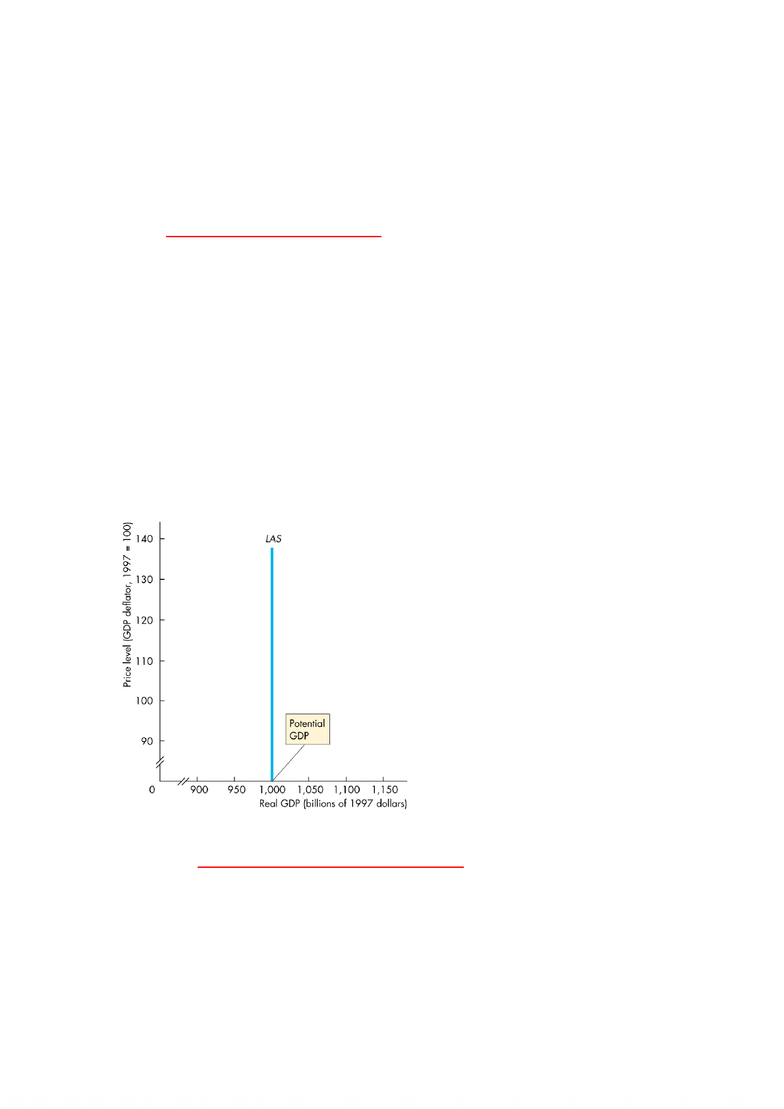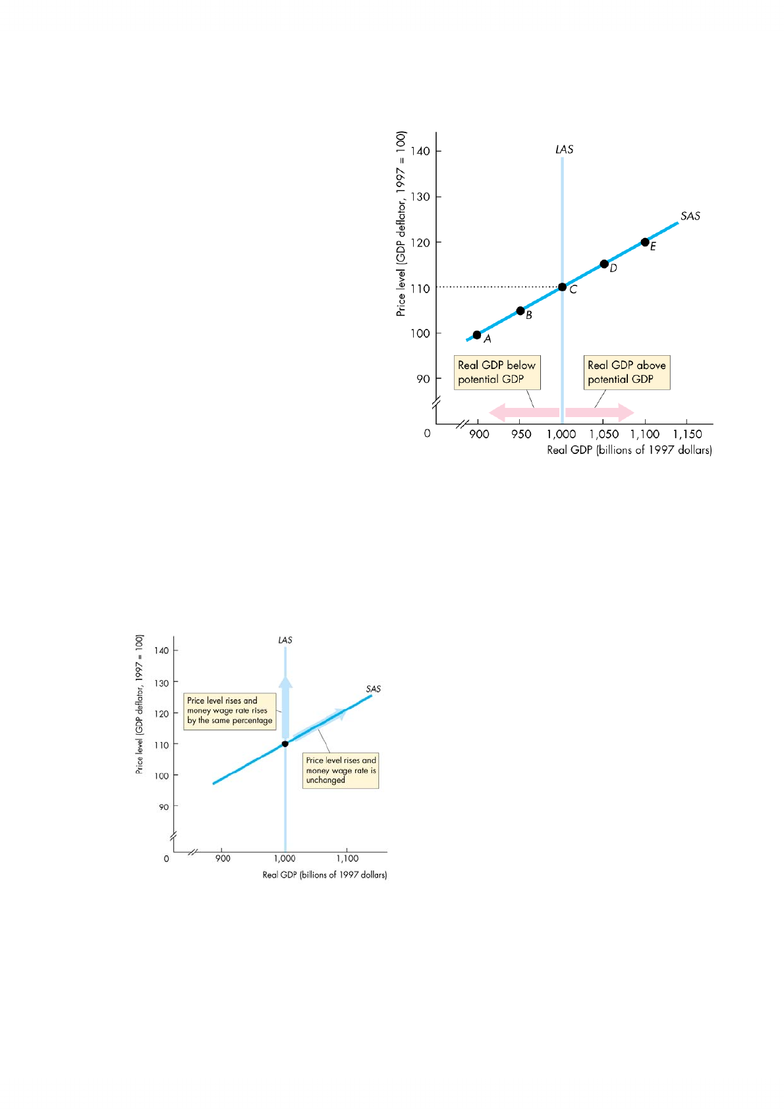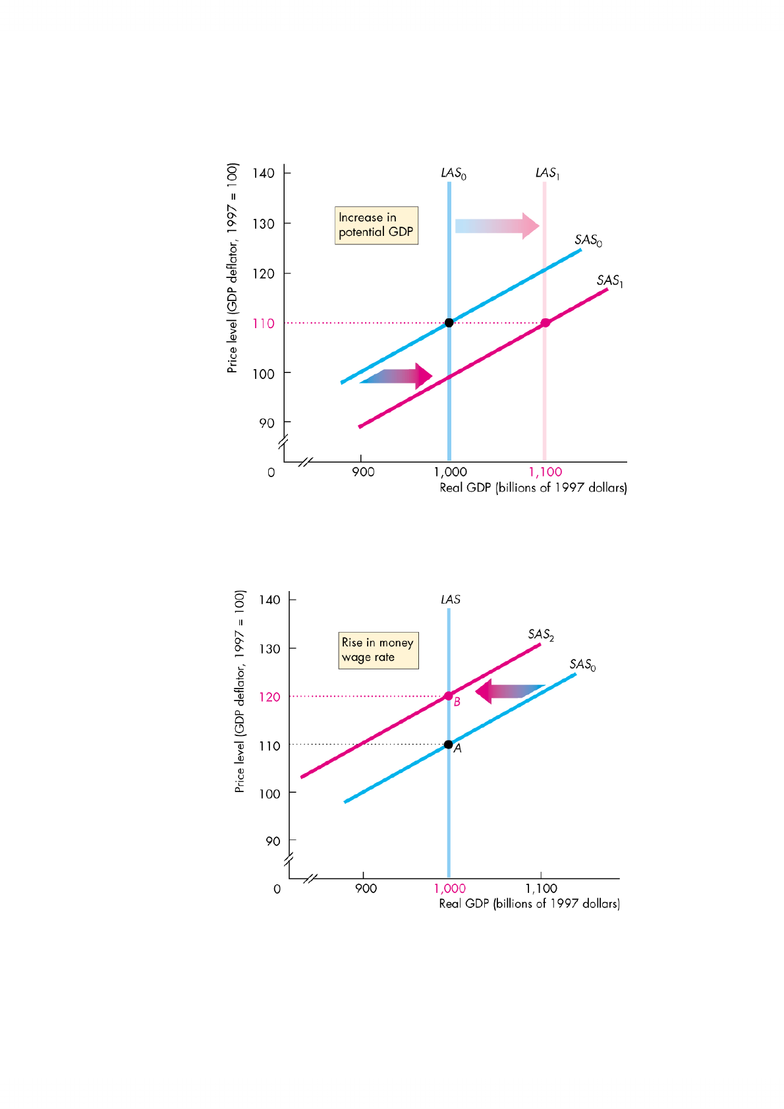# Management and Organizational Studies 2275A/B Study Guide - The Foreign Exchange, Aggregate Supply, Aggregate Demand

52 views10 pagesC
Ch
ha
ap
pt
te
er
r
2
26
6
Aggregate Supply and Aggregate Demand
A
Ag
gg
gr
re
eg
ga
at
te
e
S
Su
up
pp
pl
ly
y
Long-run aggregate supply is the relationship between the quantity of real
GDP supplied and the price level when the money wage rate changes in step
with the price level to achieve full employment.
o The quantity of real GDP supplied is the total quantity of goods and services,
valued in constant dollars, that firms plan to produce during a given time
period.
Potential GDP
is the quantity of GDP at full employment.
So the long-run aggregate supply curve is the relationship between the quantity
of real GDP supplied and the price level in the long run when real GDP equals
potential GDP.
The figure shows the long-run aggregate supply curve
LAS
, which is vertical at
potential GDP.
A movement along the
LAS
curve,
means that two sets of prices are
changing:
o The price level
o The money wage rate and
other resource prices
Because
both
the price level and
factor prices change, the
real factor
prices
remain constant.
For example, the real wage is
constant.
The real wage rate remains at the
level that achieves full employment
of labour.
The short-run aggregate supply curve is the relationship between the
quantity of real GDP supplied and the price level in the short run when the
money wage rate, the prices of other resources, and potential GDP remain
constant.
means entire
economy
Unlock document

This preview shows pages 1-3 of the document.
Unlock all 10 pages and 3 million more documents.The figure shows a short-run aggregate supply curve.
The short-run aggregate
supply curve (
SAS
) is upward
sloping.
At a price level of 100, the
quantity of real GDP supplied
is \$900 billion.
At a price level of 110, the
quantity of real GDP supplied
is \$1,000 billion, which equals
potential GDP.
At a price level of 120, the
quantity of real GDP supplied
is \$1,100 billion, which
exceeds potential GDP.
The
SAS
curve slopes upward
because, when the price level rises with a constant money wage rate, the real
wage falls.
With a lower real wage, firms make a larger profit by producing a larger output.
The figure shows the effect of price level changes on the
LAS
curve and the
SAS
curve.
A rise in both the price level and the
money wage rate that maintains full
employment brings a movement along
the
LAS
curve.
The real wage rate remains constant.
A rise in the price level at a constant
money wage rate brings a change in
employment and real GDP and a
movement along the
SAS
curve.
Long-run aggregate supply changes
when potential GDP changes.
And potential GDP changes for three
reasons:
o A change in the full-employment quantity of labour
o A change in the quantity of capital
Unlock document

This preview shows pages 1-3 of the document.
Unlock all 10 pages and 3 million more documents.When potential GDP increases, both the
LAS
curve and the
SAS
curve shift
rightward in the figure.
When the money wage rate rises, the
SAS
curve shifts leftward but the
LAS
curve remains unchanged in the figure below.
pivot point
no change in the
price level
notice LAS does
not shift
Unlock document

This preview shows pages 1-3 of the document.
Unlock all 10 pages and 3 million more documents.